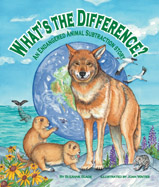Alignment to Standards for MN1 1.1.2.3 Recognize the relationship between counting and addition and subtraction. Skip count by 2s, 5s, and 10s.
1 1.1.3.1.1 many living and nonliving things are made of parts and that if a part is missing or broken, the system wont work
1 1.2.2.1 Represent real-world situations involving addition and subtraction basic facts, using objects and number sentences.
1 1.2.2.3 Use number sense and models of addition and subtraction, such as objects and number lines, to identify the missing number in an equation such as:
1 1.2.2.4 Use addition or subtraction basic facts to represent a given problem situation using a number sentence.
1 1.4.1.1.1 Describe and sort animals into groups in many ways, according to their physical characteristics and behaviors.
2 2.1.2.1 Use strategies to generate addition and subtraction facts including making tens, fact families, doubles plus or minus one, counting on, counting back, and the commutative and associative properties. Use the relationship between addition and subtraction to
2 2.1.2.2 Demonstrate fluency with basic addition facts and related subtraction facts.
2 2.1.2.4 Use mental strategies and algorithms based on knowledge of place value and equality to add and subtract two-digit numbers. Strategies may include decomposition, expanded notation, and partial sums and differences.
2 2.1.2.5 Solve real-world and mathematical addition and subtraction problems involving whole numbers with up to 2 digits.
2 2.1.2.6 Use addition and subtraction to create and obtain information from tables, bar graphs and tally charts.
2 2.2.1.1 simple number patterns involving repeated addition or subtraction, skip counting and arrays of objects such as counters or tiles. Use patterns to solve problems in various contexts.
2 2.2.2.1 Understand how to interpret number sentences involving addition, subtraction and unknowns represented by letters. Use objects and number lines and create real-world situations to represent number sentences.
2 2.2.2.2 Use number sentences involving addition, subtraction, and unknowns to represent given problem situations. Use number sense and properties of addition and subtraction to find values for the unknowns that make the number sentences true.
2 2.4.1.1.1 Describe and sort plants into groups in many ways, according to their physical characteristics and behaviors.
3 3.4.1.1.2 Identify common groups of plants and animals using observable physical characteristics, structures and behaviors.
5 5.4.2.1.2 Explain what would happen to a system such as a wetland, prairie or garden if one of its parts were changed.
5 5.4.4.1.1 Humans change environments in ways that can be either beneficial or harmful to themselves and other organisms. Give examples of beneficial and harmful human interaction with natural systems. For example: Recreation, pollution, wildlife management.
K 0.4.1.1.1 Observe and compare plants and animals.
K K.1.1.1 a number can be used to represent how many objects are in a set or to represent the position of an object in a sequence.
K K.1.1.4 Find a number that is 1 more or 1 less than a given number.
K K.1.2.1 find the sums and differences of numbers between 0 and 10.

Back to Standards Page